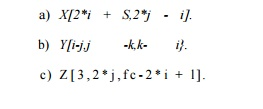Home | | Compiler Design | Affine Array Indexes

# Affine Array Indexes

1 Affine Accesses 2 Affine and Nonaffine Accesses in Practice 3 Exercises for Section 11.4

Affine Array Indexes

1 Affine Accesses

2 Affine and Nonaffine Accesses in Practice

3 Exercises for Section 11.4

The focus of this chapter is on the class of affine array accesses, where each array index is expressed as affine expressions of loop indexes and symbolic constants. Affine functions provide a succinct mapping from the iteration space to the data space, making it easy to determine which iterations map to the same data or same cache line.

Just as the affine upper and lower bounds of a loop can be represented as a matrix-vector calculation, we can do the same for affine access functions. Once placed in the matrix-vector form, we can apply standard linear algebra to find pertinent information such as the dimensions of the data accessed, and which iterations refer to the same data.

1. Affine Accesses

We say that an array access in a loop is affine if

1.          The bounds of the loop are expressed as affine expressions of the sur-rounding loop variables and symbolic constants, and

2.          The index for each dimension of the array is also an affine expression of surrounding loop variables and symbolic constants.

Example 1 1 . 1 6 :  Suppose i and j are loop index variables bounded by affine expressions.  Some examples of affine array accesses are Z[i], Z[i + j + 1], Z, Z[i, i], and Z[2*i +1,3* j — 10].  If n is a symbolic constant for a loop nest, then Z[S * n, n — j] is another example of an affine array access. However, Z[i * j] and Z[n * j] are not affine accesses.

Each affine array access can be described by two matrices and two vectors. The first matrix-vector pair is the B and b that describe the iteration space for the access, as in the inequality of Equation (11.1). The second pair, which we usually refer to as F and f, represent the function(s) of the loop-index variables that produce the array index(es) used in the various dimensions of the array access.

Formally, we represent an array access in a loop nest that uses a vector of index variables i by the four-tuple T = (F,f,B>b); it maps a vector i within the boundsExample 11 . 17: In Fig. 11.18 are some common array accesses, expressed in matrix notation.  The two loop indexes are i and j, and these form the vector i. Also, X, Y, and Z are arrays with 1, 2, and 3 dimensions, respectively. The first access, A[i - 1], is represented by a 1 x 2 matrix F and a vector f of length 1. Notice that when we perform the matrix-vector multiplication and add in the vector f, we are left with a single function, which is exactly the formula for the access to the one-dimensional array X.  Also notice the third access, Y[j,j + 1], which, after matrix-vector multiplication and addition, yields a pair of functions,  (J,j + 1).  These are the indexes of the two dimensions of the array access.

Finally, let us observe the fourth access K[l,2] . This access is a constant, and unsurprisingly the matrix F is all 0's. Thus, the vector of loop indexes, i, does not appear in the access function.2. Affine and Nonaffine Accesses in Practice

There are certain common data access patterns found in numerical programs that fail to be affine. Programs involving sparse matrices are one important example. One popular representation for sparse matrices is to store only the nonzero elements in a vector, and auxiliary index arrays are used to mark where a row starts and which columns contain nonzeros. Indirect array accesses are used in accessing such data. An access of this type, such as is a nonaffine access to the array X. If the sparsity is regular, as in banded matrices having nonzeros only around the diagonal, then dense arrays can be used to represent the subregions with nonzero elements. In that case, accesses may be affine.

Another common example of nonaffine accesses is linearized arrays. Pro-grammers sometimes use a linear array to store a logically multidimensional object. One reason why this is the case is that the dimensions of the array may not be known at compile time. An access that would normally look like Z[i,j] would be expressed as Z[i * n + j], which is a quadratic function. We can convert the linear access into a multidimensional access if every access can be decomposed into separate dimensions with the guarantee that none of the components exceeds its bound. Finally, we note that induction-variable analy-ses can be used to convert some nonaffine accesses into affine ones, as shown in Example 11.18.3. Exercises for Section 11.4

Exercise 11.4.1 : For each of the following array accesses, give the vector f and the matrix F that describe them.  Assume that the vector of indexes i is i, j , . . . , and that all loop indexes have affine limits.Study Material, Lecturing Notes, Assignment, Reference, Wiki description explanation, brief detail
Compilers : Principles, Techniques, & Tools : Optimizing for Parallelism and Locality : Affine Array Indexes |